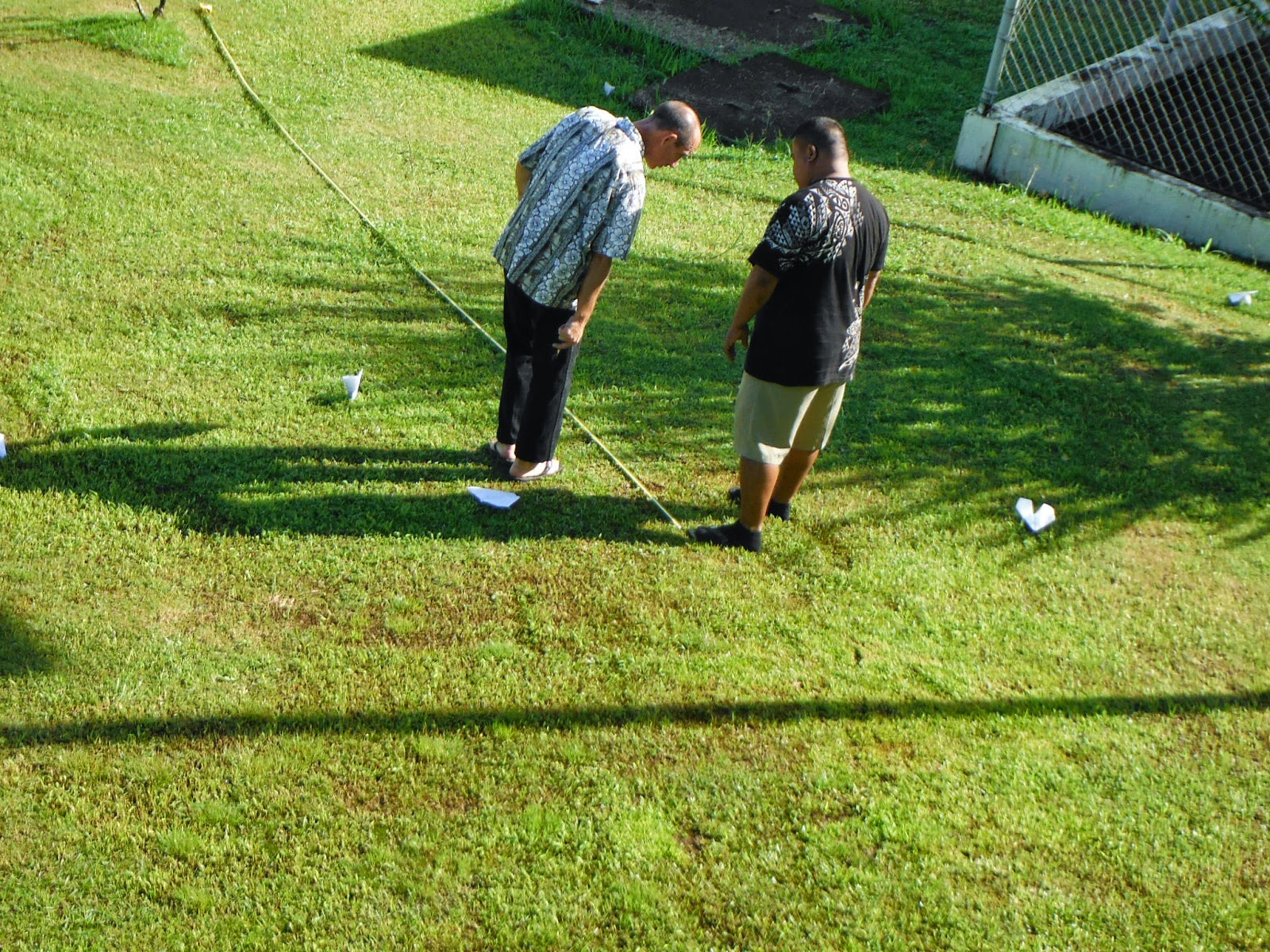## Posts

Showing posts from December, 2014

### Assessing learning in introductory statisticsMS 150 Statistics has for the past three years utilized a modified curriculum based on a proposed outline. The three course level student learning outcomes for MS 150 Statistics are:

Perform basic statistical calculations for a single variable up to and including graphical analysis, confidence intervals, hypothesis testing against an expected value, and testing two samples for a difference of means.Perform basic statistical calculations for paired correlated variables.Engage in data exploration and analysis using appropriate statistical techniques including numeric calculations, graphical approaches, and tests.
The first two outcomes involve basic calculation capabilities of the students and are assessed via an item analysis of the final examination. 76 students in three sections took the final examination.

Twenty-one questions on the final examination required the students to perform basic statistical calculations on a small sample. Based on the item analysis, 80.2% of the items were …

### Assessing learning in physical scienceproposed outline for SC 130 Physical Science includes the following three course level student learning outcomes:
Explore physical science systems using scientific methodologiesGenerate mathematical models for physical science systems and use appropriate mathematical techniques and concepts to obtain quantitative solutions to problems in physical science.Demonstrate basic communication skills by working in groups on laboratory experiments and by writing up the result of experiments, including thoughtful discussion and interpretation of data, in a formal format using spreadsheet and word processing software.
The first learning outcome requires that the students be able to explore physical science systems using scientific methodologies. For SC 130 Physical Science this exploration would be framed by the theme of mathematical models that underlie physical science systems. This, in turn, serves the general education program learning outcome, "3.5 Perform experiments that use scienti…

### Site Swap Notation in Physical ScienceDaniel Kahneman in Thinking, Fast and Slow noted that the remembering mind rates experiences using a peak-end rule. Although I had not known this particular fact when I designed laboratory 15 five years ago, I had always shared George M. Cohan's belief that one should "always leave them laughing when you say goodbye."

A class that ends on a pleasant activity makes for better memories looking back on the course. SC 130 Physical Science Laboratory 15 is both fun and yet is also a chance to introduce a mathematical model that is very different.

Laboratory fifteen in physical science sought to push the boundaries on the mathematical box for the students. In laboratory one a quote from Freeman Dyson was used to start a journey through the mathematical models that explain physical systems. Dyson calculated how an electron ought to behave. Later someone went into a laboratory and the electron behaved as predicted by the mathematical model.

In laboratory two a linear model predi…